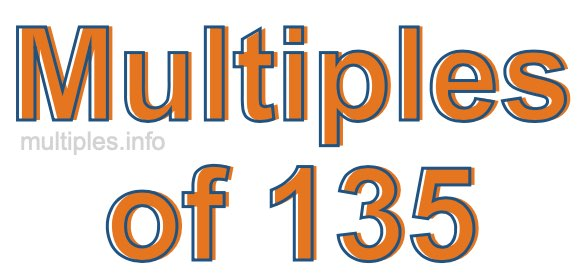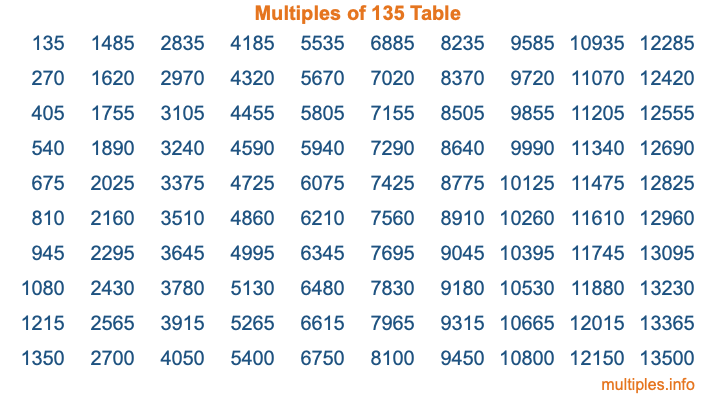Multiples of 135Welcome to the Multiples of 135 page. Here we will first teach you everything you will ever need to know about the multiples of 135, and then give you a study guide summary of everything we taught you to make sure you remember it all. Use this page to look up facts and learn information about the multiples of 135. This page will make you a multiples of one hundred thirty-five expert!

Definition of Multiples of 135
Multiples of 135 are all the numbers that when divided by 135 equal an integer. Each of the multiples of 135 are called a multiple. A multiple of 135 is created by multiplying 135 by an integer.

Therefore, to create a list of multiples of 135, you start with 1 multiplied by 135, then 2 multiplied by 135, then 3 multiplied by 135, and so on for as long as you want. Thus, the list of the first five multiples of 135 is 135, 270, 405, 540, and 675. To see a larger list of multiples of 135, see the printable image of Multiples of 135 further down on this page. We also have a category where you can choose any nth multiple of 135.

Multiples of 135 Checker
The Multiples of 135 Checker below checks to see if any number of your choice is a multiple of 135. In other words, it checks to see if there is any number (integer) that when multiplied by 135 will equal your number. To do that, we divide your number by 135. If the the quotient is an integer, then your number is a multiple of 135.

Is  a multiple of 135?

Least Common Multiple of 135 and ...
A Least Common Multiple (LCM) is the lowest multiple that two or more numbers have in common. This is also called the smallest common multiple or lowest common multiple and is useful to know when you are adding our subtracting fractions. Enter one or more numbers below (135 is already entered) to find the LCM.

Check out our LCM Calculator if you need more details about the Least Common Multiple or if you need the LCM for different numbers for adding and subtraction fractions.

nth Multiple of 135
As we stated above, 135 is the first multiple of 135, 270 is the second multiple of 135, 405 is the third multiple of 135, and so on. Enter a number below to find the nth multiple of 135.

th multiple of 135

Multiples of 135 vs Factors of 135
135 is a multiple of 135 and a factor of 135, but that is where the similarities end. All postive multiples of 135 are 135 or greater than 135. All positive factors of 135 are 135 or less than 135.

Below is the beginning list of multiples of 135 and the factors of 135 so you can compare:

Multiples of 135: 135, 270, 405, 540, 675, etc.

Factors of 135: 1, 3, 5, 9, 15, 27, 45, 135

As you can see, the multiples of 135 are all the numbers that you can divide by 135 to get a whole number. The factors of 135, on the other hand, are all the whole numbers that you can multiply by another whole number to get 135.

It's also interesting to note that if a number (x) is a factor of 135, then 135 will also be a multiple of that number (x).

Multiples of 135 vs Divisors of 135
The divisors of 135 are all the integers that 135 can be divided by evenly. Below is a list of the divisors of 135.

Divisors of 135: 1, 3, 5, 9, 15, 27, 45, 135

The interesting thing to note here is that if you take any multiple of 135 and divide it by a divisor of 135, you will see that the quotient is an integer.

Multiples of 135 Table
Below is an image of the first 100 multiples of 135 in a table. The table is in chronological order, column by column. The first column has the first ten multiples of 135, the second column has the next ten multiples of 135, and so on.The Multiples of 135 Table is also referred to as the 135 Times Table or Times Table of 135. You are welcome to print out our table for your studies.

Negative Multiples of 135
Although not often discussed or needed in math, it is worth mentioning that you can make a list of negative multiples of 135 by multiplying 135 by -1, then by -2, then by -3, and so on, to get the following list of negative multiples of 135:

-135, -270, -405, -540, -675, etc.

Multiples of 135 Summary
Below is a summary of important Multiples of 135 facts that we have discussed on this page. To retain the knowledge on this page, we recommend that you read through the summary and explain to yourself or a study partner why they hold true.

There are an infinite number of multiples of 135.

A multiple of 135 divided by 135 will equal a whole number.

135 divided by a factor of 135 equals a divisor of 135.

The nth multiple of 135 is n times 135.

The largest factor of 135 is equal to the first positive multiple of 135.

135 is a multiple of every factor of 135.

135 is a multiple of 135.

A multiple of 135 divided by a divisor of 135 equals an integer.

135 divided by a divisor of 135 equals a factor of 135.

Any integer times 135 will equal a multiple of 135.

Multiples of a Number
Here you can get the multiples of another number, all with the same attention to detail as we did for multiples of 135 on this page.

Multiples of
Multiples of 136
Did you find our page about multiples of one hundred thirty-five educational? Do you want more knowledge? Check out the multiples of the next number on our list!